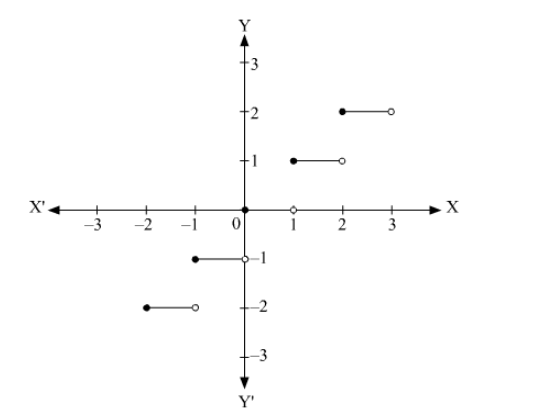# The set of points of discontinuity

Question:

The set of points of discontinuity of f(x) = [x] is ___________.

Solution:

The graph of f(x) = [x] is shown below.It can be seen that, the function f(x) = [x] is discontinuous at all integral values of i.e. x ∈ Z

Thus, the set of points of discontinuity of f(x) = [x] is the set of integers i.e. Z.

The set of points of discontinuity of f(x) = [x] is __the set of integers i.e. Z___.